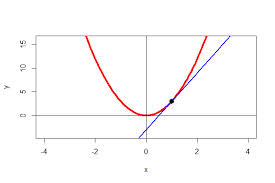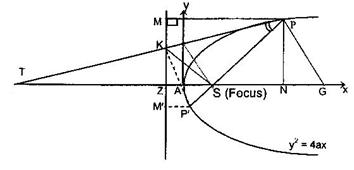×#### Thank you for registering.

One of our academic counsellors will contact you within 1 working day.

Click to Chat

1800-1023-196

+91-120-4616500

CART 0

• 0

MY CART (5)

Use Coupon: CART20 and get 20% off on all online Study Material

ITEM
DETAILS
MRP
DISCOUNT
FINAL PRICE
Total Price: Rs.

There are no items in this cart.
Continue Shopping```Tangent to a Parabola

Table of Content

Equation of Tangent to a Parabola in point form

Tangent in terms of m

Equation of the Tangents from an External Point

Chord of Contact

Point of intersection of tangents

Related ResourcesParabola is an important topic of IIT JEE Mathematics syllabus. Tangent to a parabola is an important head under parabola and it often fetches some questions in elite exams like the JEE. Hence, students are advised to prepare this topic well.

A line touching the parabola is said to be a tangent to the parabola provided it satisfies certain conditions. If we have a line y = mx + c touching a parabola y2 = 4ax, then c = a/m. Similarly, the line y = mx + c touches the parabola x2 = 4ay if c = -am2.

The line x cos c + y sin c = p touches the parabola y2 = 4ax if  a sin2c + p cos c = 0.

Remark:

In case, the equation of the parabola is not in standard form, then for the condition of tangency one must first try to eliminate one variable quanity out of x and y by solving the equations of straight line and the parabola and then use the condition B2 = 4AC for the derived quadratic equation.

Equation of Tangent to a Parabola in point form

Let P(x1, y1) and Q(x2, y2) be two neighbouring points on the parabola y2 = 4ax. Then the equation of the line joining P and Q is y – y1 = (y2 - y1) / (x2 - x1) (x – x1) …… (1)

Since, points P and Q lie on the parabola, we have

y12 = 4ax1 …… (2)

y22 = 4ax2 …… (3)

From the above two equations we have,

y22 – y12 = 4a(x2 – x1)

⇒ (y2 - y1)/(x2 - x1) = 4a/(y1 + y2)Equation of chord PQ (i.e. equation (1) becomes):

y – y1 = 4a/(y1 + y2 ) (x – x1) …… (4)

Our aim is to find the equation of tangent at point P. For that, let point Q approach point P i.e. x2 → x1 and y2 → y1.

y – y1 = 4a/(2y1) (x – x1)

⇒ yy1 = 2a (x1 + x) (using equation (2))

This is the required equation of the tangent to the parabola y2 – 4ax at P(x1, y1).

Note:

The angle between the tangents drawn to the two parabolas at the point of their intersection is defined as the angle of intersection of two parabolas.

There can be various forms of equations of tangents to a parabola. We discuss these forms one by one:

Tangent in terms of m

Suppose that the equation of a tangent to the parabola y2 = 4ax … (i)

is y = mx + c. … (ii)

The abscissae of the points of intersection of (i) and (ii) are given by the equation (mx + c)2 = 4ax. But the condition that the straight line (ii) should touch the parabola is that it should meet the parabola in coincident points

⇒ (mx – 2a)2 = m2c2 … (iii)

⇒ c = a/m. … (iv)

Hence, y = mx + a/m is a tangent to the parabola y2 = 4ax, whatever be the value of m.

Equation (mx + c)2 = 4ax now becomes (mx – a/m)2 = 0.

⇒ x = a/m2 and y2 = 4ax ⇒ y = 2a/m.

Thus the point of contact of the tangent y = mx + a/m is (a/m2, 2a/m).

Hence the various forms of tangents are listed below:

yy1 = 2a(x + x1) at the point (x1, y1)

y = mx + a/m at (a/m2, 2a/m), provided m ≠ 0

ty = x + at2 at (at2, 2at).

The points of intersection of the tangents at the point t1 and t2 is (at1t2, a(t1 + t2)).

y = mx - am2 is a tagnent to the parabola x2 = 4ay at (2am, am2) for all values of m.

Some key points:

Angle between tangents at two points P(at12, 2at1) and Q(at22, 2at2) on the parabola y2 = 4ax is

?θ = tan-1|(t2 - t1)/(1 + t1t2)|

The orthocentre of the triangle formed by the three tangents to a parabola lies on the directrix.

It can be noted that the geometric mean of the x-coordinates of P and Q i.e. the term at1t2 is also the x-coordinate of the point of intersection of tangents at P and Q on the parabola.

Illustration:

Find the condition that the line y = mx + c may touch the Parabola y2 = 4ax and also find its point of contact.

Solution:

Equation of Parabola is yy2 = 4ax …… (1)

Slope of tangent at any point is

dy/dx=2a/y = m (say) …… (2)

⇒ y = 2a/m

from (1), x = a/my2

⇒ point of contact is (a/my2 ,2a/m)

Equation of tangent is

y – 2a/m = m (x-a/my2 )

or y = mx + a/m

Therefore, the condition that y = mx + c touches the Parabola

yy2 = 4ax is c = a/m.

Illustration:

Find the equation of normal to the Parabola yy2 = 4ax, having slope m.

Solution:

Slope of tangent at any point is

dy/dx = 2a/y

Slope of normal at that point is

-y/2a = m (say)

⇒ Point of contact of a normal having slope ‘m’ with the Parabola

yy2 = 4ax is (amy2, – 2am)

So, equation of normal at this point is

y + 2am = m (x – amy2)

or y = mx – 2am – amy3.

Illustration:

If the line 2x + 3y = 1 touches the Parabola yy2 = 4ax, find the length of its latus rectum.

Solution:

Equation of any tangent to yy2 = 4ax is

y = mx + a/m ⇒ my2x – my + a = 0.

Comparing it with the given tangent 2x + 3y – 1 = 0, we find

my2/2 = (-m)/3 = a/(-1) ⇒ m = (-2)/3, a = m/3 = -2/9.

Hence the length of the latus rectum.

= 4a = 8/9, ignoring the negative sign for length.

Illustration:

One the parabola yy2 = 4ax, three points E, F, G are taken so that their ordinates are in G.P. Prove that the tangents at E and G intersect on the ordinate of F.

Solution:

Let the points E, F, G be (at1y2, 2at1), (at2y2, 2at2), (at3y2, 2at3) respectively. Since the ordinates of these points are in G.P., t22 = t1t3. tangents at E and G are t1y = x + at12 and t3y = x + at32. Eliminating y from these equation, we get x = at1t3 = at22. Hence the point lies on the ordinates of F.

Illustration:

Prove that the area of the triangle formed by three points on a parabola is twice the area of the triangle formed by the tangents at these points.The intersection of the tangents, at these points, are the points
(at1, t2, a(t1 + t2)}, {at2t3, a(t2 + t3)}, {at3 t1, a(t3 + 1)}.
The area of the Δ formed by these points=1/2 a2(t1 – t2) (t2 – t3) (t3 – t1).

Equation of the Tangents from an External Point

Let y = mx + a/m be any tangent to y2 = 4ax passing through the point (x1, y1).

Then, we have

y1 = mx + a/m or m2x1 – m1 + a = 0

If m1 and m2 are to roots of (i) then

m1 + m2 = y1/x1 and m1m2 = a/x1

Also the two tangents are y = m1x + a/m1 , and y = m2x + a/m2

⇒ Their combined equation is

(y – m1x – a/m1 ) (y – m2x – a/m2 ) = 0

On solving this we get

(y2 – 4ax) (y12 – 4ax1) = [yy1 – 2a (x + x1)]2

⇒ SS1 = T2 Where S = y2 – 4ax, S1 = y12 – 4x1

Let y2 = 4ax be the equation of a parabola and (x1, y1) an external point P. Then, equation of the tangents is given by

SS1 = T2, where S = y2 – 4ax, S1 = y12 – 4ax1, T = yy1 – 2a(x + x1).

If the tangents from the external point (x1, y1) touch the parabola at P and Q, then PQ is the chord of contact of the tangents.

Illustration:

Prove that through any given point (x1, y1) there pass, in general, two tangents to the parabola y2 = 4ax.

Solution:

The equation to any tangent is y = mx + a/m. …… (1)

If this passes through the fixed point (x1, y1), we have

y1 = mx1 + a/m, i.e. m2x1 – m y1 + a = 0. …… (2)

This is a quadratic equation and gives two values of m (real or imaginary). Corresponding to each value of m we have, two different tangents. The roots of (2) are real and different if y12 – 4ax1 > 0, i.e. If the point (x1, y1) lies outside the curve. The roots are equal, if y12 – 4ax1 = 0 i.e. if the point (x1, y1) lies on the curve. In this case the two tangent merge into one. The two roots are imaginary if y12 – 4ax1 < 0, i.e. if the point (x1, y1) lies within the curve.

Chord of Contact

The chord joining the points of contact of the tangents on the parabola from an external point is called the chord of contact.

Let the tangent drawn from the point P(x1, y1) touch Parabola at Q(x2, y2) and R(x3, y3) then QR is the chord of contact of the point P(x1, y1) withrespect to y2= 4ax.

The equation of tangents at Q and R are

yy2 = 2a(x + x2) …… (1)

yy3 = 2a(x + x3) …… (2)

Since (ii) and (iii) pass through (x1, y1) so we have

y1y2 = 2a(x1 + x2) …… (3)

y1y3 = 2a(x1 + x3) …… (4)

From (ii) and (iv) we find that the points Q(x2, y2) and R(x3, y3) lie on yy1 = 2a (x2+ x1), which being of first degree in x and y represents a straight line. Hence the equation of the chord of contact of P(x1, y1) is

yy1 = 2a (x + x1) and is of the form T = 0.

Equation of the chord of contact of the tangents drawn from a point (x1, y1) to the parabola y2 = 4ax is T = 0, i.e. yy1 – 2a(x + x1) = 0.

Point of intersection of tangents

The locus of point of intersection of tagent to the parabola y2 = 4ax with angle between them as θ is given by y2 – 4ax = (a + x)2 tan2θ.

If this angle θ = 0° or 180°, then the locus is y2 - 4ax = 0 which is actually the given parabola itself.

but, if θ = 90°, then the locus is x + a = 0 which is the equation of the directrix of the parabola.

Note:

The equation of the chord of the parabola y2 = 4ax with mid point (x1, y1) is T = S1.

Illustration:

Find the equation of the chord of the parabola y2 = 12x which is bisected at the point (5, –7).

Solution:

Here (x1, y1) = (5, –7), and y2 = 12x = 4ax ⇒ a = 3.

The equation of the chord is S1 = T

or y12 – 4ax1 = yy1 – 2a(x + x1) or (–7)2 – 12.5 = y(–7) – 6 (x + 5).

Or 6x + 7y + 19 = 0.

Related Resources

For getting an idea of the type of questions asked, refer the previous year papers.

Fast Track your revision with the ultimate Revision Notes on Trigonometry.

To read more, Buy study materials of Parabola comprising study notes, revision notes, video lectures, previous year solved questions etc. Also browse for more study materials on Mathematics here.
```### Course Features

• 731 Video Lectures
• Revision Notes
• Previous Year Papers
• Mind Map
• Study Planner
• NCERT Solutions
• Discussion Forum
• Test paper with Video Solution